### 站点信息### 尼采般地抒情### 站点信息## jQuery 属性操作

jQuery 常用属性操作有三种：prop() / attr() / data() ;

### 元素固有属性值 prop()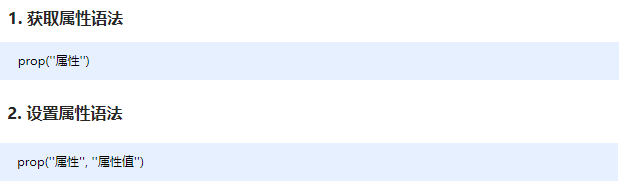### 元素自定义属性值 attr()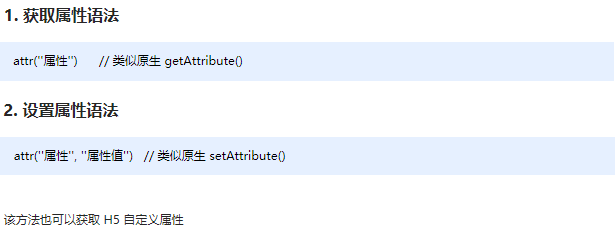### 数据缓存 data()

data() 方法可以在指定的元素上存取数据，并不会修改 DOM 元素结构。一旦页面刷新，之前存放的数据都将被移除。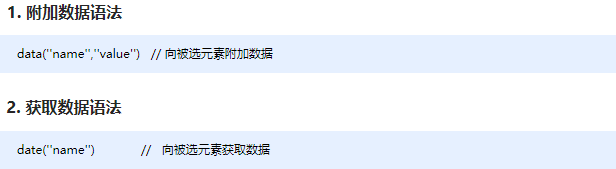``````<body>

<a href="http://www.itcast.cn" title="都挺好">都挺好</a>
<input type="checkbox" name="" id="" checked>
<div index="1" data-index="2">我是div</div>
<span>123</span>
<script>
\$(function() {
//1. element.prop("属性名") 获取元素固有的属性值
console.log(\$("a").prop("href"));
\$("a").prop("title", "我们都挺好");
\$("input").change(function() {
console.log(\$(this).prop("checked"));
});
// console.log(\$("div").prop("index"));
// 2. 元素的自定义属性 我们通过 attr()
console.log(\$("div").attr("index"));
\$("div").attr("index", 4);
console.log(\$("div").attr("data-index"));
// 3. 数据缓存 data() 这个里面的数据是存放在元素的内存里面
\$("span").data("uname", "andy");
console.log(\$("span").data("uname"));
// 这个方法获取data-index h5自定义属性 第一个 不用写data-  而且返回的是数字型
console.log(\$("div").data("index"));
})
</script>

</body>
``````

## jQuery 文本属性值

jQuery的文本属性值常见操作有三种：html() / text() / val() ; 分别对应JS中的 innerHTML 、innerText 和 value 属性。

### jQuery内容文本值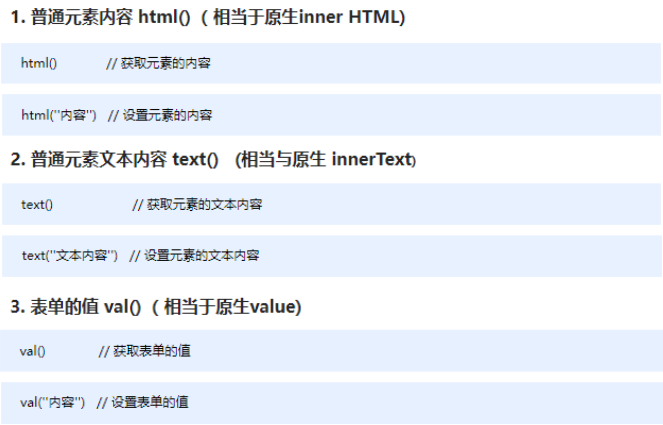``````<body>
<div>
<span>我是内容</span>
</div>
<input type="text" value="请输入内容">
<script>
// 1. 获取设置元素内容 html()
console.log(\$("div").html());
// \$("div").html("123");
// 2. 获取设置元素文本内容 text()
console.log(\$("div").text());
\$("div").text("123");
// 3. 获取设置表单值 val()
console.log(\$("input").val());
\$("input").val("123");
</script>
</body>
``````

## jQuery 元素操作

jQuery 元素操作主要讲的是用jQuery方法，操作标签的遍历、创建、添加、删除等操作。

### 遍历元素

jQuery 隐式迭代是对同一类元素做了同样的操作。 如果想要给同一类元素做不同操作，就需要用到遍历。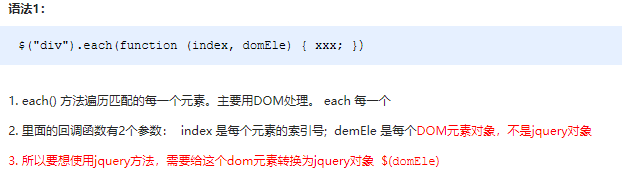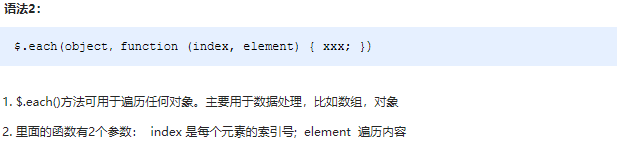``````<body>
<div>1</div>
<div>2</div>
<div>3</div>
<script>
\$(function() {
// 如果针对于同一类元素做不同操作，需要用到遍历元素（类似for，但是比for强大）
var sum = 0;
var arr = ["red", "green", "blue"];
// 1. each() 方法遍历元素
\$("div").each(function(i, domEle) {
// 回调函数第一个参数一定是索引号  可以自己指定索引号号名称
// console.log(i);
// 回调函数第二个参数一定是 dom 元素对象，也是自己命名
// console.log(domEle);  // 使用jQuery方法需要转换 \$(domEle)
\$(domEle).css("color", arr[i]);
sum += parseInt(\$(domEle).text());
})
console.log(sum);
// 2. \$.each() 方法遍历元素 主要用于遍历数据，处理数据
// \$.each(\$("div"), function(i, ele) {
//     console.log(i);
//     console.log(ele);
// });
// \$.each(arr, function(i, ele) {
//     console.log(i);
//     console.log(ele);
// })
\$.each({
name: "andy",
age: 18
}, function(i, ele) {
console.log(i); // 输出的是 name age 属性名
console.log(ele); // 输出的是 andy  18 属性值
})
})
</script>
</body>
``````

### 创建、添加、删除

jQuery方法操作元素的创建、添加、删除方法很多，则重点使用部分，如下：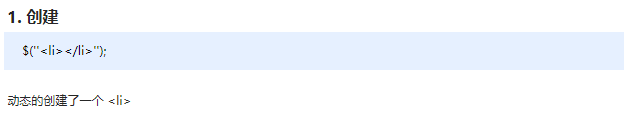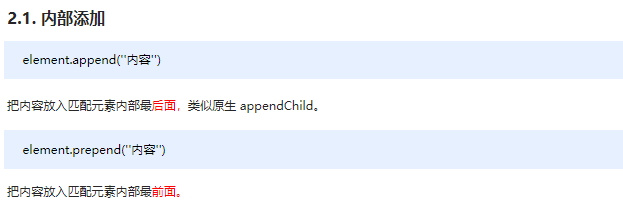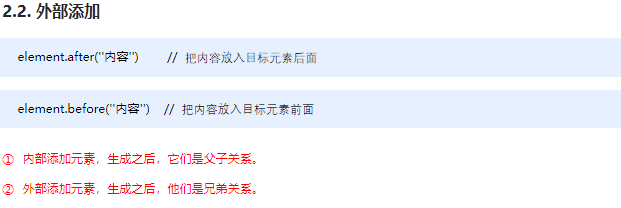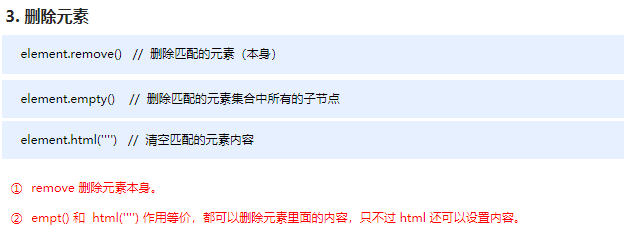``````<body>
<ul>
<li>原先的li</li>
</ul>
<div class="test">我是原先的div</div>
<script>
\$(function() {
// 1. 创建元素
var li = \$("<li>我是后来创建的li</li>");

// 2. 添加元素
//     2.1 内部添加
// \$("ul").append(li);  内部添加并且放到内容的最后面
\$("ul").prepend(li); // 内部添加并且放到内容的最前面
//  2.2 外部添加
var div = \$("<div>我是后妈生的</div>");
// \$(".test").after(div);
\$(".test").before(div);

// 3. 删除元素
// \$("ul").remove(); 可以删除匹配的元素 自杀
// \$("ul").empty(); // 可以删除匹配的元素里面的子节点 孩子
\$("ul").html(""); // 可以删除匹配的元素里面的子节点 孩子
})
</script>
</body>
``````

## jQuery 尺寸、位置操作

jQuery中分别为我们提供了两套快速获取和设置元素尺寸和位置的API，方便易用，内容如下。

### jQuery 尺寸操作

jQuery 尺寸操作包括元素宽高的获取和设置，且不一样的API对应不一样的盒子模型。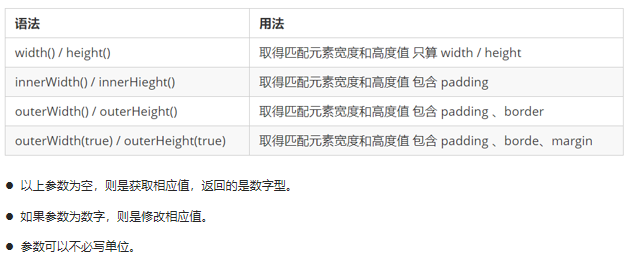``````<body>
<div></div>
<script>
\$(function() {
// 1. width() / height() 获取设置元素 width和height大小
console.log(\$("div").width());
// \$("div").width(300);
// 2. innerWidth() / innerHeight()  获取设置元素 width和height + padding 大小
console.log(\$("div").innerWidth());
// 3. outerWidth()  / outerHeight()  获取设置元素 width和height + padding + border 大小
console.log(\$("div").outerWidth());
// 4. outerWidth(true) / outerHeight(true) 获取设置 width和height + padding + border + margin
console.log(\$("div").outerWidth(true));
})
</script>
</body>
``````

### jQuery 位置操作

jQuery的位置操作主要有三个： offset()、position()、scrollTop()/scrollLeft() , 具体介绍如下: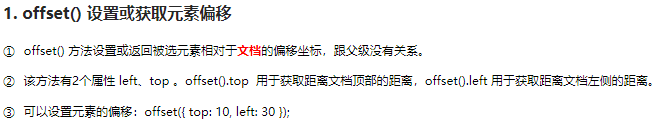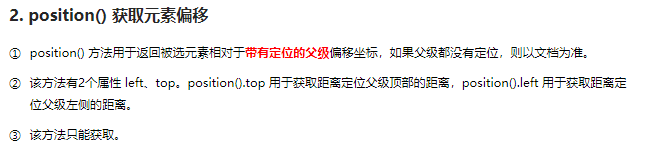``````<body>
<div class="father">
<div class="son"></div>
</div>

<div class="back">返回顶部</div>
<div class="container"></div>

<script>
\$(function() {
// 1. 获取设置距离文档的位置（偏移） offset
console.log(\$(".son").offset());
console.log(\$(".son").offset().top);
// \$(".son").offset({
//     top: 200,
//     left: 200
// });

// 2. 获取距离带有定位父级位置（偏移） position   如果没有带有定位的父级，则以文档为准
// 这个方法只能获取不能设置偏移
console.log(\$(".son").position());
// \$(".son").position({
//     top: 200,
//     left: 200
// });

// 3. 被卷去的头部
\$(document).scrollTop(100);
// 被卷去的头部 scrollTop()  / 被卷去的左侧 scrollLeft()
// 页面滚动事件
var boxTop = \$(".container").offset().top;
\$(window).scroll(function() {
// console.log(11);
console.log(\$(document).scrollTop());
if (\$(document).scrollTop() >= boxTop) {
} else {
}
});
// 返回顶部
\$(".back").click(function() {
// \$(document).scrollTop(0);
\$("body, html").stop().animate({
scrollTop: 0
});
// \$(document).stop().animate({
//     scrollTop: 0
// }); 不能是文档而是 html和body元素做动画
})
})
</script>
</body>
``````

Twikoo giscus